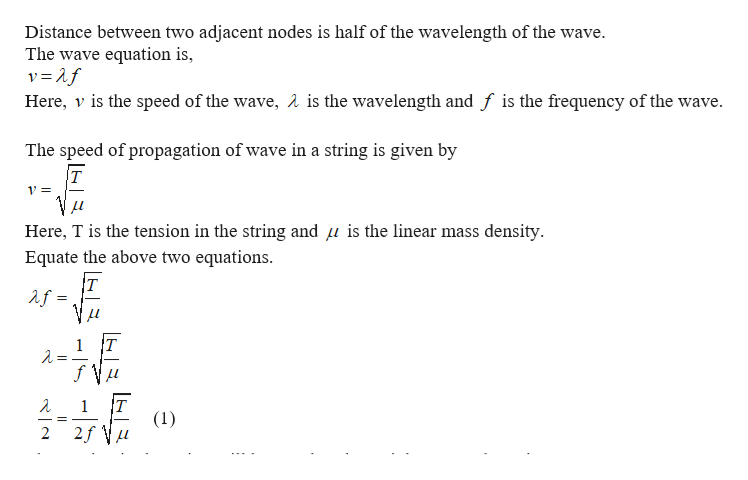# Standing Waves: You put a mass of 400 g on the string. (The string is 50 cm long and weights 12.5 g)What distance between adjacent nodes do you then expect for a frequency of 100 Hz? (Use g = 10 m/s2)not clear on how to go about this questions, as you calculate please give reason...as you always do thanks

Question

Standing Waves: You put a mass of 400 g on the string. (The string is 50 cm long and weights 12.5 g)

What distance between adjacent nodes do you then expect for a frequency of 100 Hz? (Use g = 10 m/s2)

check_circle

Step 1help_outlineImage TranscriptioncloseDistance between two adjacent nodes is half of the wavelength of the wave. The wave equation is, Here, v is the speed of the wave, A is the wavelength and f is the frequency of the wave The speed of propagation of wave in a string is given by T 1= Here, T is the tension in the string and u is the linear mass density Equate the above two equations T af T 1 1 (1) 2 fullscreen

### Want to see the full answer?

See Solution

#### Want to see this answer and more?

Solutions are written by subject experts who are available 24/7. Questions are typically answered within 1 hour.*

See Solution
*Response times may vary by subject and question.
Tagged in

### Wave Motion